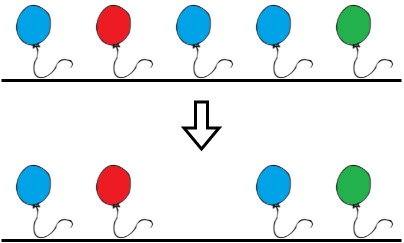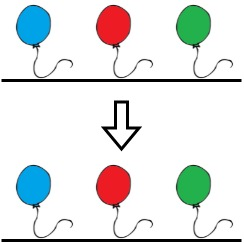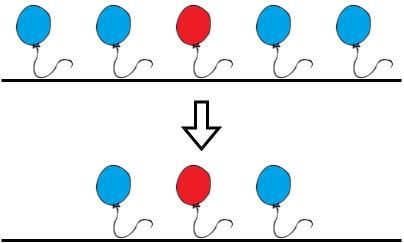# 1578. Minimum Time to Make Rope Colorful | LeetCode Solution

## 1578. Minimum Time to Make Rope Colorful

Alice has `n` balloons arranged on a rope. You are given a 0-indexed string `colors` where `colors[i]` is the color of the `ith` balloon.

Alice wants the rope to be colorful. She does not want two consecutive balloons to be of the same color, so she asks Bob for help. Bob can remove some balloons from the rope to make it colorful. You are given a 0-indexed integer array `neededTime` where `neededTime[i]` is the time (in seconds) that Bob needs to remove the `ith` balloon from the rope.

Return the minimum time Bob needs to make the rope colorful.

Example 1:```Input: colors = "abaac", neededTime = [1,2,3,4,5]
Output: 3
Explanation: In the above image, 'a' is blue, 'b' is red, and 'c' is green.
Bob can remove the blue balloon at index 2. This takes 3 seconds.
There are no longer two consecutive balloons of the same color. Total time = 3.```

Example 2:```Input: colors = "abc", neededTime = [1,2,3]
Output: 0
Explanation: The rope is already colorful. Bob does not need to remove any balloons from the rope.
```

Example 3:```Input: colors = "aabaa", neededTime = [1,2,3,4,1]
Output: 2
Explanation: Bob will remove the ballons at indices 0 and 4. Each ballon takes 1 second to remove.
There are no longer two consecutive balloons of the same color. Total time = 1 + 1 = 2.
```

Constraints:

• `n == colors.length == neededTime.length`
• `1 <= n <= 105`
• `1 <= neededTime[i] <= 104`
• `colors` contains only lowercase English letters.
Solution:

We will keep the track of previous characters i.e color of the balloon. If we get to a point where the prev balloon and the current balloon have the same color then we move till the same color and find the balloon that will take the maximum time and the total time that would be taken to burst all the balloons. At last, we will subtract the maximum time from the total time and we will add it to our answer.

If the previous balloon color is not the same as of current we will simply update the prev.

See the code for a better understanding.